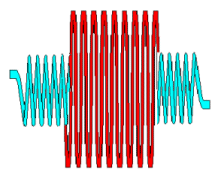Teaching Physics with the Physics Suite Edward F. Redish

Induced current in a coil

 A long narrow coil is surrounded by a short wide coil as shown in the figure at the right. The short wide coil has a diameter dS, nS turns per unit length, and a length S. It has its ends connected through a resistor of resistance R. The long narrow inner coil has a diameter dL, nL turns per unit length, and a length L. It has its ends connected across a variable power source. For each of the partial sentences below, indicate on your answer sheet whether they are correctly completed by the phrase greater than (>), less than (<), or the same as (=). If you cannot determine which is the case from the information given, indicate not sufficient information (NSI).The current through the inner coil is increased from 0 to 0.1 Amps over a period of 10 seconds in a smooth fashion according to the rule

iS(t) = (0.01 A/s) t.

1. The magnitude of the current in the short wide coil at time t = 1 s is __________ the current in that coil at time t = 5 s.
2. The magnitude of the current in the long narrow coil at time t = 1 s is __________ the current in that coil at time t = 5 s.
3. The magnitude of the current in the long narrow coil at time t = 1 s is __________ the current in the short wide coil at that same time.
4. If the long narrow coil was compressed to half its length before the current was turned on, the current in the short wide coil would be __________ it was without the compression.

Not finding what you wanted? Check the Site Map for more information.